/

### Annualisation Conventions: Tools

The Nematrian website provides a web service, MnRestateYieldOrDiscount  that converts interest or discount rates quoted using one annualisation convention to equivalent rates using a different annualisation convention. With this function, the relevant annualisation convention is defined by a parameter (taking only integral values) that if zero means continuous compounding, if positive (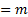) means expressed as an interest rate compounded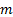times per annum and if negative (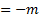) means expressed as a discount rate compoundedtimes per annum.

Usually, derivative pricing algorithms concentrate on continuously compounded rates (i.e. ‘forces’ of interest), since this simplifies the mathematical presentation. For example, if interest rates vary through time then the accumulated value after time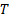of an investment of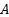now (i.e. at time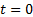), if the continuously compounded interest rate between now and then is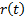at timeis: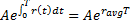where: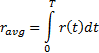This corresponds to the natural way in which we would expect to average a quantity through time. If we used other annualisation conventions then the averaging process involves more complicated mathematical formulae.

The impact of different annualisation conventions is shown in Illustratative table of annualisation conventions.

Exact computation of present values of cash flows generally requires not just information about the annualisation convention applicable to the interest rate or discount rate in question, but also precise information about when the cash flows will occur. In real life, the ‘expected’ date on which these may occur may not be their actual date of payment, e.g. the specified date may not be a business day. Moreover, if, say, coupon payments are specified as annual percentages of nominal amounts but are paid more frequently than annually, say quarterly, then it become necessary to specify how these annual amounts are apportioned between the different quarter dates. These types of conventions are usually called day count conventions.